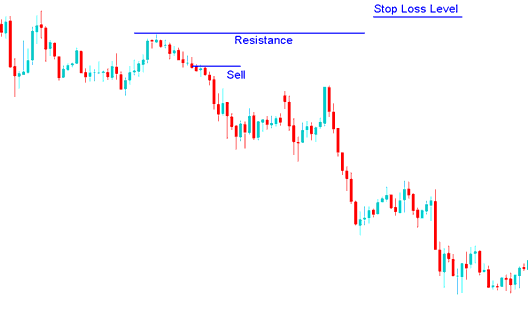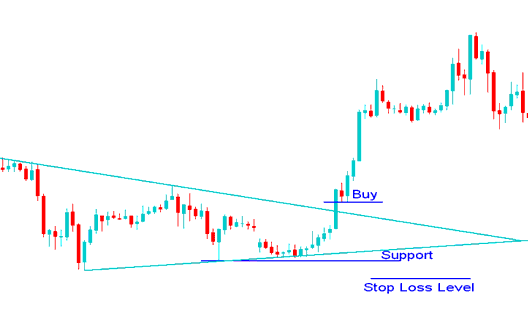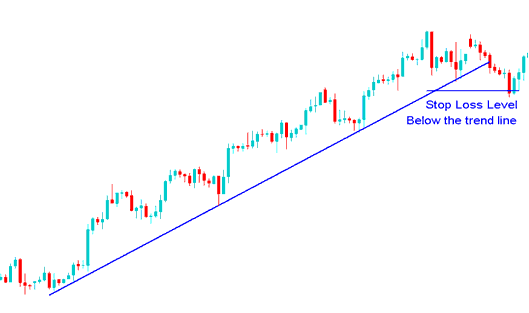# Methods of Setting Stop Loss Orders In Forex Trading

Traders using a Forex trading system must have mathematical calculations that reveal where the order must be placed.

A Forex trader can also place a stop loss order according to the technical indicators used to set these orders. Certain technical indicators use mathematical equations to calculate where the stop loss order should be set so as to provide an optimal exit point. These indicators can be used as the basis for setting these orders.

Other forex traders also place these orders according to a predetermined risk to reward ratio. This method of setting is dependent upon certain mathematical equations. For example a ratio of 50 pips stop loss can be used by a Forex trader if the trade has the potential to make 100 pips in profit; this is a risk reward ratio of 2:1

Others just use a predetermined percentage of their total trading account balance.

To set a stop loss order it is best to use one of the following methods:

## 1. Percentage of trading account balance

This is based on the percent of account balance that the trader is willing to risk.

If a currency trader is willing to risk 2% of account balance then the trader determines how far he will set the order level based on the position size that he has bought or sold.

Example:

If a trader has a \$100,000 account and is willing to risk 2%

• If the trader buys 1 contract
1 pip = \$10

Then setting at 2%

2% is \$ 2,000

2000 /10 = 200 pips

Stop loss = 200 pips

• If the trader buys 2 contracts
1 pip = \$20

Then setting at 2 %

2% is \$ 2,000

2000 /20 = 100 pips

Stop loss = 100 pips

• If the trader buys 4 contracts
1 pip = \$40

Then setting at 2 %

2% is \$ 2,000

2000 /40 = 50 pips

Stop loss = 50 pips

## 2. Setting Stop Loss using Support and Resistance Levels

Another way of setting stop loss orders is to use supports and resistance levels, on the trading charts.

Given that stop loss orders tend to congregate at key points, when one of these levels is touched by the price, others are set off, like dominos. Stop loss orders tend to accumulate just above or below the resistance or support levels, respectively.

A resistance or a support level should act like a barrier for price movement, this is why they are used to set stop losses, if this barrier is broken the price movement can go towards the opposite direction of the original currency trade, but if this barriers (support and resistance levels) are not broken the price will continue moving in the intended direction.### Stop Loss level using a resistance levelSetting order above the resistance

### Stop Loss level using a support LevelSetting order below the Support Line

## 3. Forex Trend Lines

A Forex trend line can be used to set stop losses where the order is set just below the trend line. As long as the trend line holds the trader will be able to continue making profits while at the same time set this order that will lock his profit once the trend line is broken.Setting order below the trend line

Example of where to set this order using Forex trend lines.